This document provides a brief introduction to Global Sensitivity Analysis (GSA) and it provides a workflow to perform GSA in R using the SAFE (Sensitivity Analysis For Everybody) toolbox (see References 1-2), using as an example an actuarial pricing model.

We consider both the case where the model is run in R and where it is run in a different environment (such as Excel), in the latter case we guide the user through the steps to upload the simulated model results run in Excel.

The model is an actuarial pricing model where we test the influence of variations in four inputs (i.e. Frequency Trend, Severity Trend, Exposure Trend and Loss Development Pattern) on the uncertainty of the output (Losses).

But first,

## What is Sensitivity Analysis? and why shall we use it?

Sensitivity Analysis (SA) is:

a set of mathematical techniques which investigate how uncertainty in the output of a numerical model can be attributed to variations of its input factors.

Benefits:

1. Better understanding of the model

Evaluation of model behaviour beyond default set-up

2. “Sanity check” of the model

Does the model meet the expectations (model validation)?

3. Prioritize investments for uncertainty reduction

Identify sensitive inputs for computer-intensive calibration, acquisition of new data, etc.

4. More transparent and robust decisions

Understand main impacts of uncertainty on modelling outcome and thus on decisions

## How Global Sensitivity Analysis (GSA) works

Let’s say we want to test how the uncertainty of 4 model inputs (or assumptions) influence the variability of the model output.

The input factor is any element that can be changed before running the model. In general, input factors could be equations implemented in the model, set-up choices needed for the model execution on a computer, parameters and input data.

In our model example the input factors could be continuous and discrete variables, or the distribution of an input (in which case we want to investigate how changing the distribution of that input influences the uncertainty of the output).

The output can be any variable that is obtained after the model’s execution.

Before evaluating the model, we will simulate the inputs in their range of variability and then run the model so that for each simulation all the 4 inputs vary simultaneously (Input Sampling step). For every output of interest a probability distribution is obtained, after which sensitivity analysis with the method of choice is performed, which allows to obtain a set of sensitivity indices for each output (i.e. one per input, which shows the relative influence input factors have on the output) (Reference 4).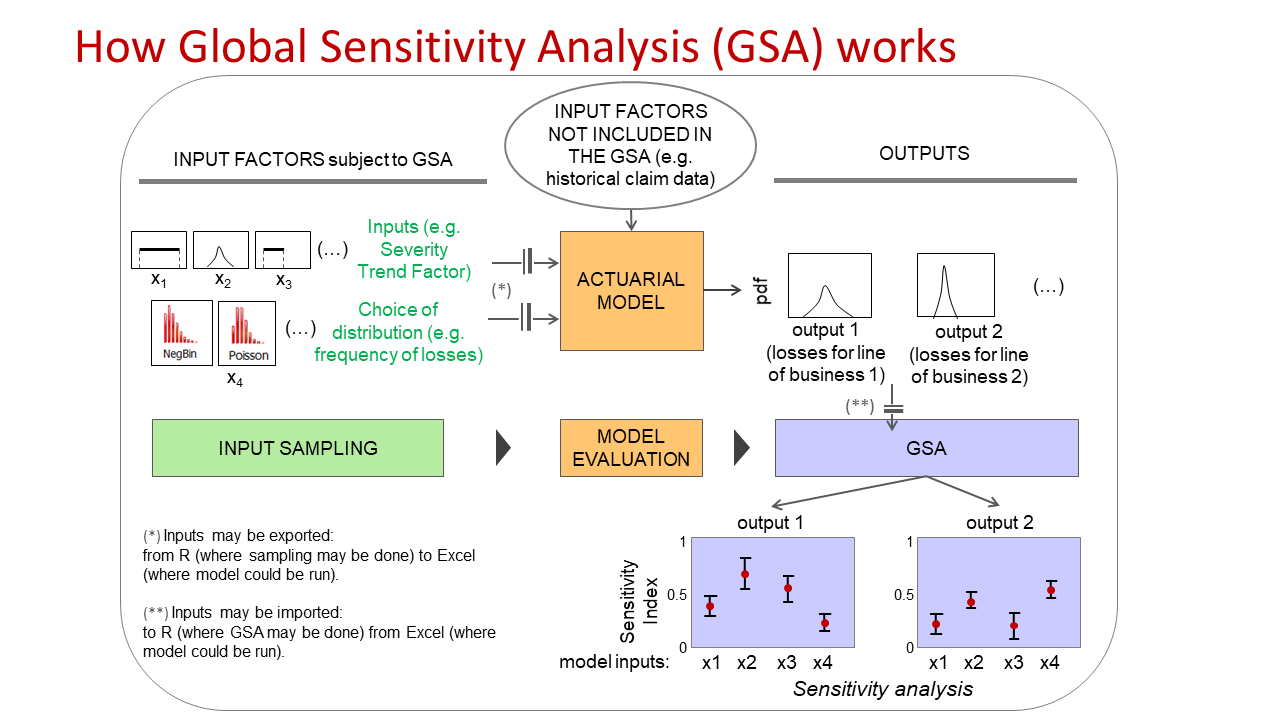## How to choose which SA method to use?

It depends on the question you want SA to answer.

In general, SA can have three purposes:

1. Ranking (or Factor Prioritization) to rank the input factors based on their relative contribution to the output variability.

2. Screening (or Factor Fixing) to identify the input factors, if any, which have a negligible influence on the output variability.

3. Mapping to determine the region of the input variability space which produces output values of interest (e.g. extreme values).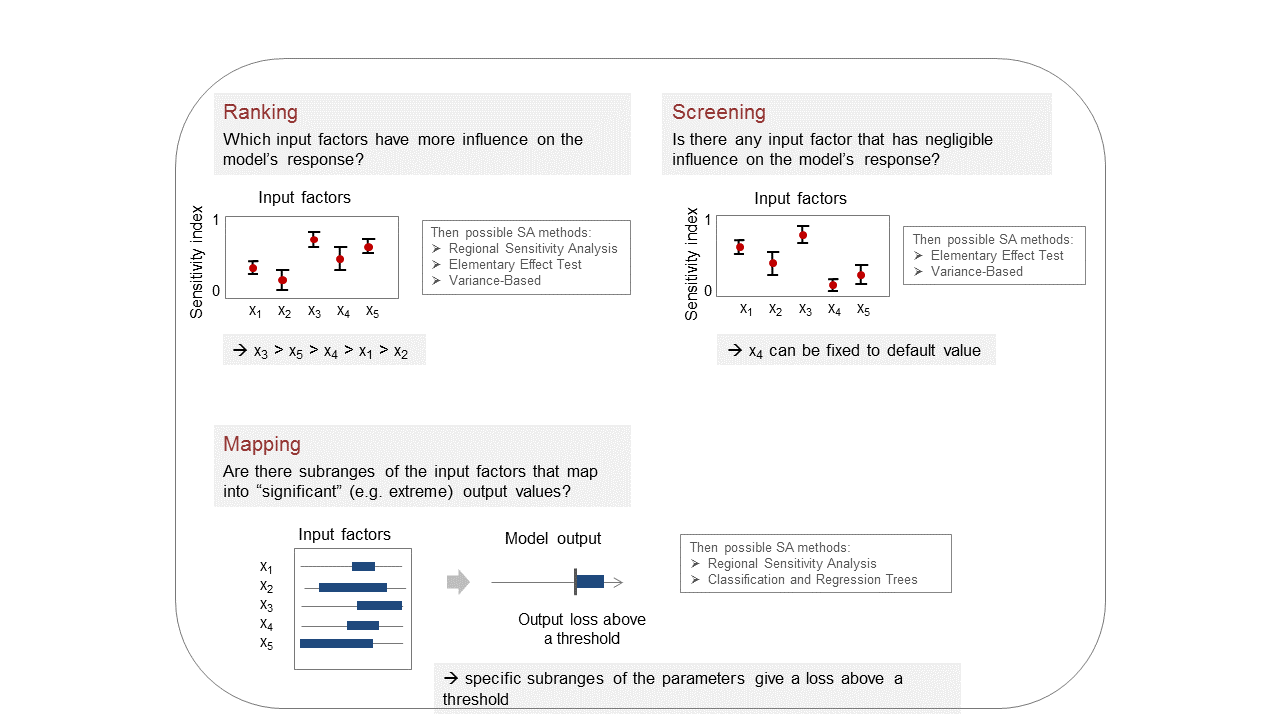## GSA workflow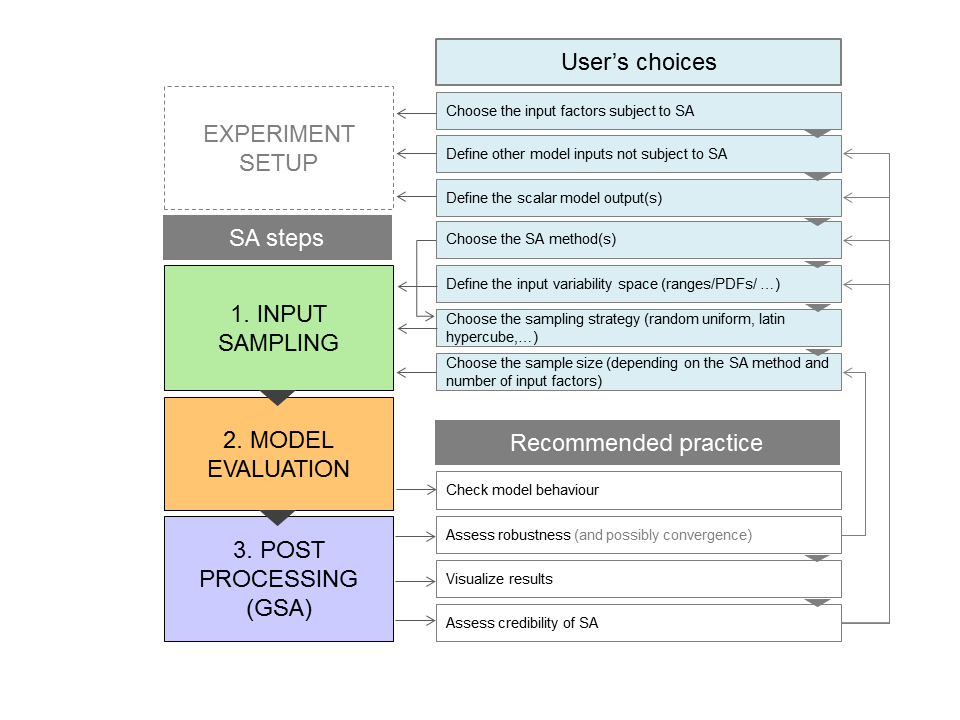There are 3 main steps in the GSA workflow:

1. Input Sampling

2. Model Evaluation

3. Post Processing (actual GSA routine)

But before starting there are a few choices one should take:

1. Choose the model input factors of which you want to investigate their influence on the model output through SA.
2. Define the other model inputs not subject to SA.
3. Define the scalar model output(s).

1. Afterwards, the Input Sampling require the following steps:
1. Decide the SA method(s) to use depending on the purpose of the analysis (e.g. ranking, screening, mapping).
2. Define the input variability space (i.e. the plausible range of values the inputs can take and their distribution).
3. Choose the sampling strategy (random uniform, Latin hypercube, …).
4. Choose the number of samples (depending on the SA method and the number of input factors).

1. Run the model (Model Evaluation)

1. Post Processing (GSA)
1. Check that the model behaviour meets the expectations, and if not check whether this is due to any bug/error in the model.
2. Assess the robustness through bootstrapping to assess if the number of samples used is sufficient (and preferably the convergence of the sensitivity indices too).
3. Visualise the results (through scatter plots, parallel coordinate plots, …)
4. Assess the credibility of the SA results (e.g. Is the impact of the varying inputs on the output as expected? Are the most influential inputs those expected? Is there any odd/unexpected behaviour? Are the confidence intervals of the sensitivity indices adequate for your purpose?)

Possibly use more than one SA method to verify the results consistency.

### Step 1: Load the packages

Install and load the packages below.

library(caTools)
library(calibrater) # Install from tar file, also available at: https://people.maths.bris.ac.uk/~mazjcr/calibrater_0.51.tar.gz
library(SAFER) # Install from zip
library(ggplot2)

The Input Sampling step can be done either in R or in Excel, if done in Excel skip to Step 5b.

### Step 2: Define input factors subject to SA

The distribution of the inputs DistrFun and their range DistrIn can be chosen by expert judgement, available data (e.g. portfolio or market data) or literature.

DistrFun  <- "unif" # Inputs distribution
DistrIn  <- list( c(0, 1), c(0, 1), c(0, 1), c(0, 1)) # Range of each input
x_labels <- c("Freq. trend","Sev. trend","Exposure trend", "Dev. pattern") # Name of inputs

### Step 3: Sample inputs space

The number of model evaluations (N) typically increases proportionally to the number of input factors (M) and will depend on the SA method chosen too. As a rule of thumb, it may require more than 10 model evaluations per input factor (M) for the most frugal methods (e.g. Elementary Effect Test) and more than 1000 model evaluations per M for the more expensive methods (e.g. Variance-Based) (see References 3-4 for further details).

SampStrategy <- "lhs" # Here the sampling strategy for All At the Time (AAT) sampling is
# Latin hypercube (another option is random uniform)
N <- 500 # Sample size
M <- length(DistrIn) # Number of inputs
X_s <- AAT_sampling(SampStrategy, M, DistrFun, DistrIn, N) # Sample inputs space
colnames(X_s) <- x_labels # Set columns names

### Step 4: Save sampled inputs to file

write.csv(X_s, file = "Input_samples.csv", row.names = FALSE)

### Step 5: Run the model

#### a) If the model is in R

Y <- actuarial_model(X_s) # Where 'actuarial_model' is your chosen model
X1 <- X_s[,1]
X2 <- X_s[,2]
X3 <- X_s[,3]
X4 <- X_s[,4]

#### b) If the model is in Excel

Run the model in Excel. Then load the file with the output simulations (one row per simulation and one column per input sampled and per simulated output).

M <- 4 # Define number of input factors (if model was run in Excel)

head(DataSA) # Display first rows to check format
##       output   X1   X2   X3  X4
## 1 0.12222604 0.25 0.25 0.50 0.2
## 2 0.68218255 1.00 0.00 0.25 0.2
## 3 0.00000000 0.25 0.50 0.50 0.0
## 4 0.01440512 1.00 0.50 0.50 0.6
## 5 0.32099952 0.00 0.50 0.75 0.6
## 6 1.86312468 0.00 1.00 0.75 0.4

### Step 6: Clean data by removing errors

If data contain NA or errors, as in this case, remove the corresponding rows.

idxn <- is.na(DataSA$output) # Get index of rows with NA Y <- DataSA$output[!idxn] # Assign to Y output without NA, do the same for Xi:
X1 <- DataSA$X1[!idxn] X2 <- DataSA$X2[!idxn]
X3 <- DataSA$X3[!idxn] X4 <- DataSA$X4[!idxn]
X <- matrix(c(X1,X2,X3,X4), nrow = length(X1), ncol = M)

### Step 7: Check model behaviour by visualising input/output samples

Use SAFER function scatter_plots to visualise inputs/output

x_labels <- c("Freq. trend","Sev. trend","Exposure trend", "Dev. pattern")
sz_tx <- 12 # Font size for plots

N <- length(Y) # Get number of samples (now without NA)
colnames(X) <- x_labels # Set column names
scatter_plots(X, Y, prnam = x_labels) + ylab("Losses (in million ?)")  +
xlab("Input value") + theme(text = element_text(size=sz_tx))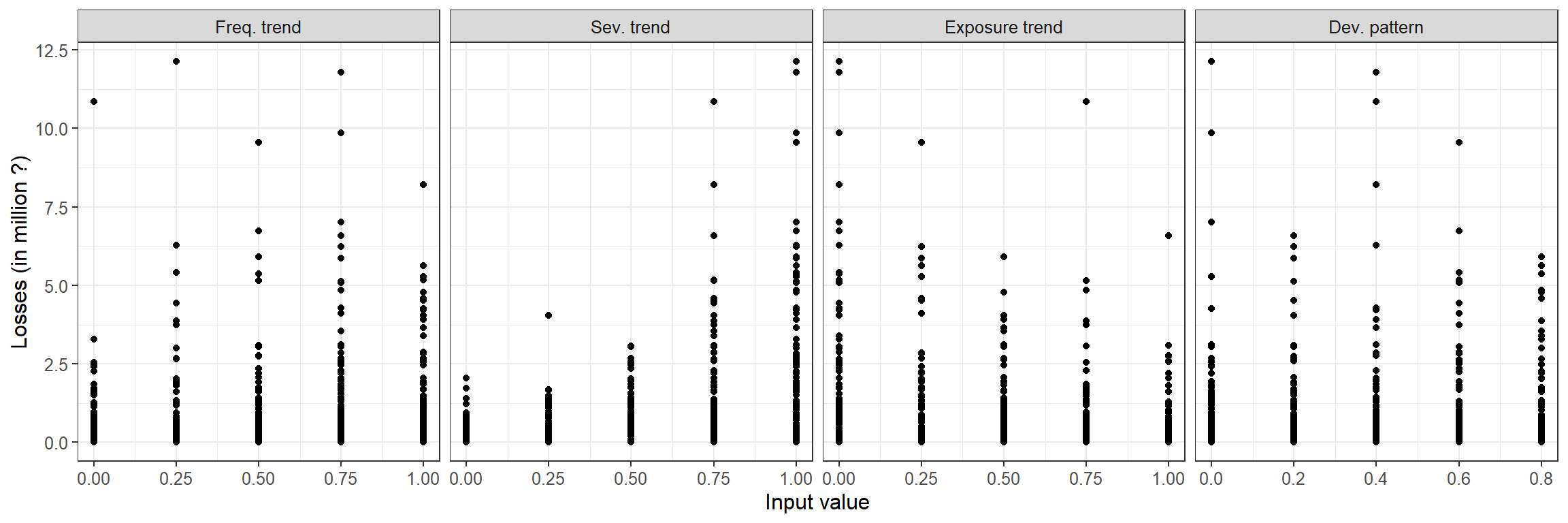Question: From these scatter plots, which input factor would you say is most influential? Why?
Are there input factors that are not influential at all?

$\begin{array}{l} -------------------------------------------------------------- \\ \\ -------------------------------------------------------------- \\ \\ -------------------------------------------------------------- \\ \end{array}$

### Step 8: Compute sensitivity indices with RSA

Let’s now apply Sensitivity Analysis: for example Regional Sensitivity Analysis (RSA), which aims at identifying regions in the inputs space corresponding to particular regions (e.g. high or low) of the output.

RSA requires to sort the output and then to spilt the output into different groups. Afterwards, we identify regions in the inputs space which produced output in each group.

So let’s divide the output into n_groups number of groups, where each group contains the same number of samples.

n_groups <- 5; # Number of groups into which the output is splitted, default = 10

flag <- 2; # where flag: statistic for the definition of the RSA index (scalar)
# flag <- 1: stat = median (default)
# flag <- 2: stat = maximum

rsa_gr <- RSA_indices_groups(X, Y, n_groups,flag)

# Outputs
mvd <- rsa_gr$stat # mvd: maximum vertical distance between CDFs (sensitivity index) (see Steps 10-11) idx <- rsa_gr$idx # idx: index which divides the output into different groups
Yk <- rsa_gr\$Yk # Yk: output limit of each group

### Step 9: Visualise input/output samples divided by group

Let’s now replot the results with the function scatter_plots where each group of inputs corresponds to a range of output (as estimated in Step 8) and is plotted with a different colour.

scatter_plots(X, Y, ngr = n_groups, prnam = x_labels) +
ylab("Losses (in million ?)") + xlab("Input value") +
theme(text = element_text(size=sz_tx))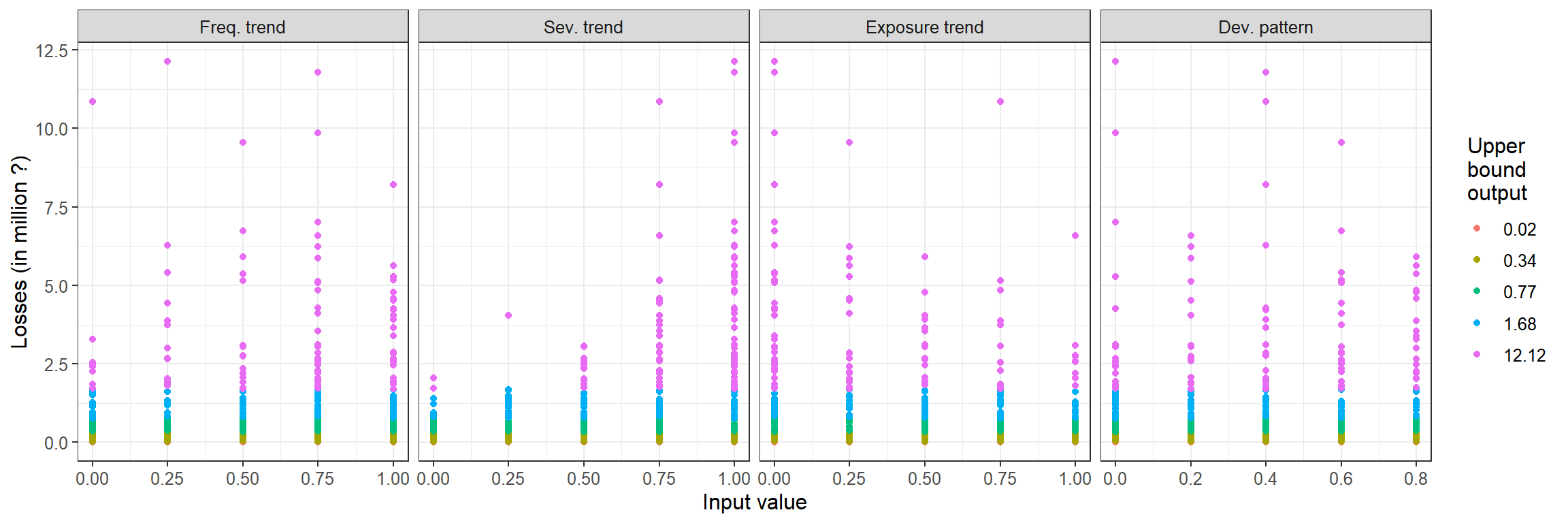### Step 10: Plot inputs CDFs

Here the CDFs of each input are plotted (where the inputs are divided among different groups depending on the range of output they produce, as in Step 8).

RSA_plot_groups(X, idx, Yk, prnam = x_labels)  + xlab("Input value") +
theme(text = element_text(size=sz_tx))